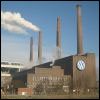14.01SC | Fall 2011 | Undergraduate

# Principles of Microeconomics

Unit 3: Producer Theory

## Productivity and Costs

« Previous | Next »

### Session OverviewEverything has a cost, and that is true for firms as well as consumers. When firms produce goods, they incur costs that vary depending on how much they are producing. In this lecture, we will analyze firms’ cost functions. Building factory infrastructure is a producer cost. Image courtesy of AndreasPraefcke on Wikipedia.

Keywords: Productivity; food production; costs; marginal costs; long run costs; short run costs.

### Session Activities

Before watching the lecture video, read the course textbook for an introduction to the material covered in this session:

• [R&T] Chapter 8, “Production and Cost.”
• [Perloff] Chapter 7, “Costs.” (optional)

### Check Yourself

#### Concept Quiz

This concept quiz covers key vocabulary terms and also tests your intuitive understanding of the material covered in this session. Complete this quiz before moving on to the next session to make sure you understand the concepts required to solve the mathematical and graphical problems that are the basis of this course.

###### Question 1

In the short run, how is the marginal cost defined?

The capital rental rate multiplied by the number of machines needed to produce one additional unit. close
The capital rental rate multiplied by the number of machines used. close
The wage rate multiplied by the amount of labor used. close
The wage rate multiplied by the number of hours needed to produce one additional unit. check

The wage rate multiplied by the amount of labor used and the capital rental rate multiplied by the number of machines used are total costs for labor and capital, not marginal costs. The capital rental rate multiplied by the number of machines needed to produce one additional unit also fails to define marginal cost, because in the short-run, capital is not variable, so it cannot be increased to produce additional goods.

###### Question 2

An isocost line is defined as combinations of labor and capital that yield the same levels of what quantity?

Cost. check
None of these. close
Profit. close
Revenue. close

An isocost line represents combinations of labor and capital that can be obtained for the same total cost.

###### Question 3

The economically efficient point of production for firms is identified by the tangency of what two objects?

An isoquant is tangent to the X axis. close
An isoquant is tangent to the budget constraint. close
An isoquant is tangent to the isocost line. check
Two isoquants are tangent to each other. close

An isoquant is tangent to the isocost line. This is the point where the ratio of marginal products of each input is equal to the ratio of prices, defining the optimal point of production for the firm. It is impossible for two isoquants to be tangent to each other, as isoquants do not cross. Whether the isoquant is tangent to the X-axis is not a relevant for firm-decision-making. It is also impossible for an isoquant to be tangent to the budget constraint, as a budget constraint is a construct used to describe the firm's problem, not the consumer's problem.

###### Question 4

For a fast-food business like McDonald's, as the business expands capital becomes less productive, and the business requires more and more labor. What does this imply about the long-run expansion path?

It depends on the specific production function. close
It is concave (i.e., the slope decreases as L increases). check
It is convex (i.e., the slope increases as L increases). close
It is linear. close

The expansion path is linear if there is no change in the relative productivity of labor and capital as the business expands. It is convex if capital becomes relatively more productive and the business requires less and less labor (e.g., a high-tech, capital-intensive business.) The specific production function is not relevant if you know how the relative productivities of inputs changes over time.

###### Question 5

What is an example of sunk costs for someone opening a law practice?

Rent on office space. close
The cost of a law school education. check
The cost of office supplies. close
Wages for paralegals. close

The cost of a law school education is a sunk cost because irrespective of future decisions, these costs can never be recouped.

« Previous | Next »

## Course Info

Fall 2011
##### Learning Resource Types
Lecture Videos
Recitation Videos
Problem Sets with Solutions
Exams with Solutions
Exams
Lecture Notes
Exam Materials
Problem Sets